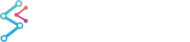﻿ Advanced Axis 3D APIs | WPF Chart DocumentationSciChart WPF 3D Charts > Axis3D APIs > Advanced Axis 3D APIs

Several Axis APIs are shared with SciChart 2D’s AxisCore type. These are summarised below:

## LabelProvider API – Full Control over Axis Labels

The LabelProvider API allows full control over the formatting of AxisCore text labels, over and above what you can achieve by setting TextFormatting.

Use the LabelProvider when you want to:

• Have fine-grained control over Axis Text or Cursor Labels, depending on numeric (or date) values.
• Display strings on Axis e.g. “Bananas”, “Oranges”, “Apples”, not “1”, “2”, “3”
• Dynamically change the Axis Textformatting as you zoom in or out.
• Dynamically change the Axis Textformatting depending on data-value.

NOTE: This is a shared API between SciChart 2D and SciChart 3D. For a full walk-through of the AxisCore LabelProvider API, including code-samples, please see Section 4.5.1.

## Full Control over Axis ticks – Tick Provider and Delta Calculator API

The TickProvider and DeltaCalculator APIs provide full control over the frequency of axis labels and gridlines, over and above what you can achieve by setting AxisBase3D.MajorDelta and MinorDelta.

NOTE: This is a shared API between SciChart 2D and SciChart 3D. For a full walk-through of the AxisCore TickProvider API, including code-samples, please see Section 4.5.2 - 4.5.3.

## Axis Data/Pixel Coordinate Conversion API

SciChart 3D provides a clean and simple API to transform 3D world coordinates to data-values and vice versa via the AxisCore.GetCurrentCoordinateCalculator() API.

#### Where World Coordinates are measured from

World coordinates are virtual coordinates in the 3D World. They are defined by Vector3 struct which comprises X,Y,Z components.All points in ‘World Coordinates’ are measured from the origin, which by default is the center floor of the Axis Cube.

All Axes are responsible for converting between data-values and world-coordinates: the XAxis converts X data-values to X-world coordinates, the YAxis converts Y data-values to Y-world coordinates and the ZAxis converts Z-data values to Z-world coordinates.

#### Getting a CoordinateCalculator instance

AxisBase3D.GetCurrentCoordinateCalculator() returns a coordinate calculator instance which is valid for the current render pass.

Note: If the AxisBase3D.VisibleRange changes, the data changes, or the SciChart3DSurface.WorldDimensions changes, then the CoordinateCalculator will give incorrect results. It is advisable to cache the Coordinate Calculator only for a short period of time, e.g. when using inside a loop.

#### Converting from Data to Pixels

Data-values are converted to pixel coordinates via the ICoordinateCalculator.GetCoordinate() method.

Converting NumericAxis3D Data to World Coordinates
Copy Code
```// Get coordinate from a NumericAxis3D
NumericAxis3D numericAxis = new NumericAxis3D();
var coordCalc = numericAxis.GetCurrentCoordinateCalculator();

// GetCoordinate expects a double value. You can cast to double if your data is integer
double worldCoordinate = coordCalc.GetCoordinate(1.23d);
```
Converting TimeSpanAxis3D Data to World Coordinates
Copy Code
```// Get coordinate from a TimeSpanAxis3D
TimeSpanAxis3D numericAxis = new TimeSpanAxis3D();
var coordCalc = numericAxis.GetCurrentCoordinateCalculator();

// GetCoordinate expects a double value. You can pass in TimeSpan.Ticks
double worldCoordinate = coordCalc.GetCoordinate(TimeSpan.FromMilliseconds(1234).Ticks);
```
Converting DateTimeAxi3D Data to World Coordinates
Copy Code
```// Get coordinate from DateTimeAxis3D
DateTimeAxis3D dateTimeAxis = new DateTimeAxis3D();
var coordCalc = numericAxis.GetCurrentCoordinateCalculator();

// GetCoordinate expects a double value. You can pass in DateTime.Ticks
double worldCoordinate = coordCalc.GetCoordinate(new DateTime(2015, 01, 01).Ticks);
```
Converting NumericAxis3D Pixels to World Coordinates
Copy Code
```// Get data-value from a coordinate with NumericAxis3D
NumericAxis3D numericAxis = new NumericAxis3D();
var coordCalc = numericAxis.GetCurrentCoordinateCalculator();

// GetDataValue expects a world coordinate and returns double
// You can cast the return value if your data is integer
double worldCoord = 12.34;
double dataValue = coordCalc.GetDataValue(worldCoord);
```
Converting TimeSpanAxis3D Pixels to Data
Copy Code
```// Get data-value from a coordinate with TimeSpanAxis
TimeSpanAxis3D timeSpanAxis = new TimeSpanAxis3D();
var coordCalc = timeSpanAxis.GetCurrentCoordinateCalculator();

// GetDataValue expects a world coordinate and returns double
// You need to wrap the double in a TimeSpan to complete the conversion
double worldCoord = 12.34;
double dataValueAsDouble = coordCalc.GetDataValue(worldCoord);
TimeSpan dataValue = new TimeSpan((long) dataValueAsDouble);
```
Converting DateTimeAxis3D Pixels to Data
Copy Code
```// Get data-value from a coordinate with DateTimeAxis
DateTimeAxis3D dateTimeAxis = new DateTimeAxis3D();
var coordCalc = dateTimeAxis.GetCurrentCoordinateCalculator();

// GetDataValue expects a pixel coordinate and returns double
// You need to wrap the double in a DateTime to complete the conversion
double worldCoord = 12.34;
double dataValueAsDouble = coordCalc.GetDataValue(worldCoord);
DateTime dataValue = new DateTime ((long)dataValueAsDouble);
```